Name:    Unit 1 Practice Test

Matching

Match each item with the correct statement below.
 a. absolute zero e. mass b. Kelvin temperature scale f. significant figure c. Celsius temperature scale g. precision d. weight h. accuracy

1.

closeness to true value

2.

narrowness of range of measurements

3.

the quantity of matter an object contains

4.

the lowest point on the Kelvin scale

5.

the SI scale for temperature

6.

the force of gravity on an object

7.

the non-SI scale for temperature

Multiple Choice
Identify the choice that best completes the statement or answers the question.

8.

What is the result of adding 2.5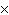10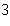and 3.510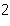?
 a. 2.910b. 6.010c. 2.910d. 6.010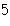9.

Which group of measurements is the most precise? (Each group of measurements is for a different object.)
 a. 2 g, 3 g, 4 g c. 1.2 g, 1.4 g, 1.3 g b. 2.0 g, 3.0 g, 4.0 g d. 1 g, 3 g, 5 g

10.

In the measurement 0.503 L, which digit is the estimated digit?
 a. 5 b. 3 c. the 0 immediately to the left of the 3 d. the 0 to the left of the decimal point

11.

Which of the following volumes is the smallest?
 a. one decaliter b. one liter c. one milliliter d. one deciliter

12.

What is the volume of a salt crystal measuring 2.4410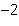m by 1.410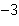m by 8.410m?
 a. 2.910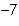mc. 2.910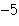mb. 2.910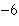md. 2.910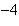m13.

What is the temperature of absolute zero measured inC?
 a. –373C b. –273C c. –173C d. –73C

14.

Which temperature scale has no negative temperatures?
 a. Celsius b. Fahrenheit c. Joule d. Kelvin

15.

Which of the following mass units is the largest?
 a. 1 cg b. 1 dg c. 1 mg d. 1 dag

16.

The weight of an object ____.
 a. is the same as its mass c. is not affected by gravity b. depends on its location d. is always the same

17.

What is the temperature –34C expressed in kelvins?
 a. 139 K b. 207 K c. 239 K d. 339 K

18.

If the temperature changes by 100 K, by how much does it change inC?
 a. 0C b. 37C c. 100C d. 273C

19.

A train travels at a speed of 30 miles per hour. If 1 mile = 1.6 kilometers, how fast is the train traveling in kilometers per minute?
 a. 0.4 km/min c. 0.8 km/min b. 0.6 km/min d. 1.0 km/min

20.

Density is found by dividing ____.
 a. mass by volume c. mass by area b. volume by mass d. area by mass

21.

What is the density of an object having a mass of 8.0 g and a volume of 25 cm?
 a. 0.32 g/cmb. 2.0 g/cmc. 3.1 g/cmd. 200 g/cm22.

How is 4.12 x 104 written in standard decimal notation?
 a. 41200 b. 0.000412 c. 4120 d. 0.0412

23.

Which is the correct measurement for location marked by the arrow?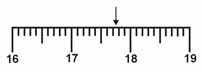a. 17.076 c. 17.76 b. 17.8 d. 17.7

24.

The accepted value is 1.43. Which correctly describes this student’s experimental data?
 Trial Measurement 1 1.29 2 1.93 3 0.88
 a. accurate but not precise c. both accurate and precise b. precise but not accurate d. neither accurate nor precise

25.

Which term is described as the amount of matter in an object?
 a. density c. mass b. volume d. length

26.

Which term describes the amount of mass contained per unit volume?
 a. mass c. length b. temperature d. density

27.

An object has a mass of 26.94 grams and a volume of 2.568 cubic centimeters. What material is it likely to be made of?
 Substance iron gold silver copper Density (g/cm3) 7.874 19.32 10.49 8.92
 a. Iron c. Silver b. Gold d. Copper

28.

The product of 2 x 104 cm and 4 x 10–12 cm, expressed in scientific notation is ____.
 a. 8 x 10–7 cm c. 8 x 10–8 cm b. 6 x 10–8 cm d. 8 x 10–48 cm

29.

A theory is a ____.
 a. proposed explanation for an observation b. well-tested explanation for a broad set of observations c. summary of the results of many observations d. procedure used to test a proposed explanation

30.

The accepted value for the density of iron is 7.87 g/cm3. A student records the mass of a 20.00-cm3 block of iron as 153.8 grams. What is the percent error for the density measurement?
 a. 19.4% c. 2.29% b. 7.69% d. 2.34%

31.

A bottle contains 3.100 mL of a liquid. The total mass of the bottle and the liquid together is 6.300 g. The mass of the empty bottle is 4.240 g. What is the density of the liquid?
 a. 0.665 g/mL c. 1.505 g/mL b. 1.368 g/mL d. 2.032 g/mL

32.

Which of the following observations is quantitative?
 a. The liquid turns blue litmus paper red. b. The liquid boils at 100°C. c. The liquid tastes bitter. d. The liquid is cloudy.

33.

Which of the following observations is qualitative?
 a. A chemical reaction was complete in 2.3 seconds. b. The solid had a mass of 23.4 grams. c. The pH of a liquid was 5. d. Salt crystals formed as the liquid evaporated.

34.

A statement that can be tested experimentally is a
 a. variable. b. model. c. generalization. d. hypothesis.

35.

All of the following are examples of units except
 a. mass. b. kilometer. c. gram. d. ounce.

36.

Which of these is not an SI base unit?
 a. kilogram b. second c. liter d. Kelvin

37.

The symbols for units of length in order from largest to smallest are
 a. m, cm, mm, km. c. km, mm, cm, m. b. mm, m, cm, km. d. km, m, cm, mm.

38.

The unit m3 measures
 a. length. b. area. c. volume. d. time.

39.

A volume of 1 milliliter is equivalent to
 a. 1 cubic centimeter. c. 1 liter. b. 1 gram. d. 10–1 cubic decimeters.

40.

A change in the force of gravity on an object will affect its
 a. mass. b. density. c. weight. d. kinetic energy.

41.

The relationship between the mass m of a material, its volume V, and its density D is
 a. D = mV. b. D = V/m. c. D = m/V. d. D = m + v.

42.

The density of pure diamond is 3.5 g/cm3. What is the volume of a diamond with a mass of 0.25 g?
 a. 0.071 cm3 b. 0.875 cm3 c. 3.75 cm3 d. 14 cm3

43.

10 000 milliliters is equivalent to
 a. 1 hectoliter. c. 1 centiliter. b. 1 dekaliter. d. 1 deciliter.

44.

0.25 g is equivalent to
 a. 250 kg. b. 250 mg. c. 0.025 mg. d. 0.025 kg.

45.

The number of grams equal to 0.5 kg is
 a. 0.0005 b. 0.005 c. 500 d. 5000

46.

How many minutes are in 1 week?
 a. 168 min b. 1440 min c. 10 080 min d. 100 800 min

47.

If 1 inch equals 2.54 cm, how many centimeters equal 1 yard?
 a. 0.0706 cm b. 14.2 cm c. 30.5 cm d. 91.4 cm

48.

A numerical result is said to have good precision if
 a. it agrees closely with an accepted value. b. repeated measurements agree closely. c. it has a small number of significant figures. d. it is a large whole number.

49.

How is the measurement 0.000 065 cm written in scientific notation?
 a. 65 x 10–6 cm c. 6.5 x 10–6 cm b. 6.5 x 10–5 cm d. 6.5 x 10–4 cm

50.

The average distance between the Earth and the moon is 386 000 km. Expressed in scientific notation, this distance is written as
 a. 386 x 103 km. c. 3.9 x 105 km. b. 39 x 104 km. d. 3.86 x 105 km.

51.

When 1.92 x 10–6 kg is divided by 6.8 x 102 mL, the quotient equals
 a. 2.8 x 10–4 kg/mL. c. 2.8 x 10–8 kg/mL. b. 2.8 x 10–5 kg/mL. d. 2.8 x 10–9 kg/mL.

52.

The result of dividing 107 by 10–3 is
 a. 10–4 b. 102.5 c. 104 d. 1010

53.

What is the result of multiplying 2.510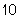by 3.510?
 a. 8.7510c. 8.7510b. 8.7510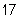d. 8.7510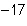54.

How is 2.97 x 10-5 written in standard decimal notation?
 a. 0.000297 b. 29700 c. 2.97e-05 d. 297000

55.

The result of multiplying 105 by 10–2 is
 a. 10–3 b. 103 c. 107 d. 10-10

True/False
Indicate whether the statement is true or false.

56.

If you wear glasses already, you do not need to wear safety goggles.

57.

When moving from a small unit, such as grams, to a larger unit, such as kilograms, the numerical value will increase.

58.

When recording a measurement using an instrument that has markings that represent the one hundreds place, you must record your answer to the closest ones place.

59.

A temperature of 55°F is approximately equivalent to 13°C.

60.

A measurement of 5.685 x 10-6 is equivalent to 0.0000005685.